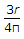# Civil Engineering - UPSC Civil Service Exam Questions

31.

If the time required for 60% consolidation of a remoulded soil sample of clay with single drainage is t, then what is the time required to consolidate the same sample of clay with the same degree of consolidation but with double drainage ?

 A. 4 t B. 2 t C. t/2 D. t/4

Explanation:

No answer description available for this question. Let us discuss.

32.

Which one of the following is the correct expression for the versine (h) of a curve ?(Where l = length of rail on the curve portion and r = radius of curvature)

 A.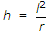B.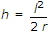C.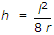D.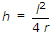Explanation:

No answer description available for this question. Let us discuss.

33.

The principle of virtual work states that the virtual work is zero for

 A. a body moving with constant linear velocity B. a body rotating with constant angular velocity C. a body in equilibrium D. a body moving with constant linear acceleration

Explanation:

No answer description available for this question. Let us discuss.

34.

A uniform beam of span l carries a uniformly distributed load W per unit length as shown in the figure. The supports are at a distance of x from either end. What is the condition for the maximum bending moment in the beam to be as small as possible?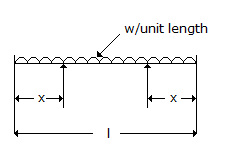A. x = 0.107 l B. x = 0.207 l C. x = 0.237 l D. x = 0.25 l

Explanation:

No answer description available for this question. Let us discuss.

35.

The distance of the centroid of a semi-circle of radius r from its base is

 A.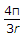B.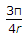C.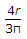D.